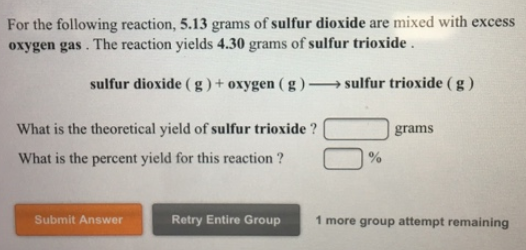# Problem: For the following reaction, 5.13 grams of sulfur dioxide are mixed with excess oxygen gas. The reaction yields 4.30 grams of sulfur trioxide. sulfur dioxide (g) + oxygen (g) → sulfur trioxide (g) What is the theoretical yield of sulfur trioxide? What is the percent yield for this reaction ?

###### FREE Expert Solution###### Problem Details

For the following reaction, 5.13 grams of sulfur dioxide are mixed with excess oxygen gas. The reaction yields 4.30 grams of sulfur trioxide.

sulfur dioxide (g) + oxygen (g) → sulfur trioxide (g)

What is the theoretical yield of sulfur trioxide?

What is the percent yield for this reaction ?